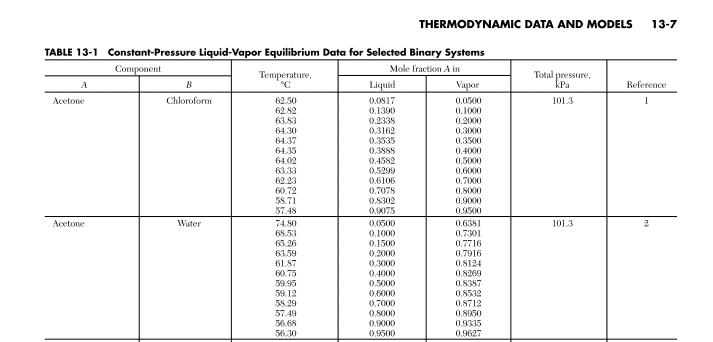# Binary VLE

## Homework Statement

This is not homework. I am having trouble interpreting this data. I don't see how it satisfies a conservation of mass. As the temperature is increasing, how is acetone increasing its mole fraction in both phases? If the mole fraction of acetone increases in one phase, then shouldn't it decrease in the other phase? Mole fraction is a conserved quantity right?

## Homework EquationsPerry Handbook

## The Attempt at a Solution

see 

TSny
Homework Helper
Gold Member
Hi swmmr1928. I'm fairly sure "mole fraction of A in liquid phase" means the ratio nAl/(nAl+nCl) where n refers to number of moles and the superscript "l" refers to liquid phase. "A" is for acetone and "C" is for chloroform.

Likewise, "mole fraction of A in vapor state" meansn nAv/(nAv+nCv) where "v" refers to vapor state.

With this definition, you can see that the sum of the mole fractions of A in liquid and vapor phases does not need to remain constant as temperature changes.

Here's a little exercise. (Numbers are totally made up!) Consider a system of 10 moles of A and 10 moles of C initially with both substances entirely liquid. Slowly raise the temperature until some of the liquid begins to vaporize. Suppose at temperature T1 we find

nAl = 9.9 moles nAv = 0.1 moles (Note total is still 10 moles)
nCl = 9.98 moles nCv = 0.02 moles

Note that the vapor is richer in acetone because acetone is more volatile than chloroform.

Now we raise the temperature to T2 and find

nAl = 6.0 moles nAv = 4.0 moles
nCl = 8.5 moles nCv = 1.5 moles

Calculate the mole fractions of A in the liquid and vapor phases for these two temperature. Do you find that the mole fractions of A in both phases decrease with increase in temperature?

Chestermiller
Mentor
An easy way to get a feel for this is to consider two liquids that form an ideal solution when mixed, and whose vapors form an ideal gas solution in the gas phase. Your two substances do not quite do this, but that won't detract from the analysis for the ideal case.

Let xa and xb represent the mole fractions of the two species in the liquid phase, and let ya and yB represent the mole fractions of the two species in the gas phase. Let P represent the total pressure, which in your situation is a constant. Let pa (T) be the equilibrium vapor pressure of pure a at temperature T, and pb (T) be the equilibrium vapor pressure of pure b at temperature T. Then for the ideal situation above,

P ya = pa xa
P yb = pb xb

If you add these two equations together, you get:

P = pa xa + pb (1 - xa)

Solving for xa, you then get:

xa= (P - pb) / (pa - pb)

For a given temperature, this equation gives the mole fraction of a in the liquid phase. The mole fraction of a in the gas phase is then given by:

ya = pa xa / P

In many cases, the ratio of the equilibrium vapor pressures pa / pb is fairly insensitive to temperature (i.e., assuming nearly matching heats of vaporization of the pure substances). This is called the assumption of constant "relative volatility." If we make this approximation, and apply it to the first two equations, we obtain:

ya/(1 - ya) = xa/ (1 - xa)(pa / pb)

According to this equation, ya would increase monotonically with xa under the constant relative volatility approximation.